Skip to the navigation links

# Matplotlib conversion

CIAO 4.11 is the first release that includes the Python Matplotlib plotting package (it includes version 2.2.3). There are many guides and tutorials online to using Matplotlib, including the Matplotlib usage guide, Jake VanderPlas' Visualization with Matplotlib, and the Python 4 Astronomers guide. This page concentrates on helping ChIPS users convert to using Matplotlib.

Although the overall concepts are similar between the two systems, the following guide is not going to cover all the functionality of ChIPS or Matplotlib. It is split up into the following sections:

## Loading the module

Matplotlib provides several interfaces to control the appearance of plots. This guide will focus on the "pyplot" interface, and will assume it has been loaded using the following statement:

>>> from matplotlib import pyplot as plt


Note that, unlike the ChIPS module, Matplotlib is not automatically loaded for you in either the Sherpa or ChIPS applications, and so must be manually imported.

## Displaying plots

When using IPython - either directly via the ipython command or with the CIAO-provided wrappers, chips and sherpa - the following commands (which use the Matplotlib plot command to plot a set of points) does not appear to create a display:

>>> plt.plot([10, 20, 30], [5, -2, 12])
[<matplotlib.lines.Line2D at 0x7f3ea21eedd8>]


This is because of "technical reasons" involving event loops, but rather than bore you with the details, here are several possible solutions.Note

Matplotlib commands that "create" something on the plot, such as the plot call, will return something (in this case a list of objects). The return values can be used to control the appearance of the objects, and so you will often want to save these values in a script, as will be described below. In an interactive session it may not be worth the effort.

### Using show

The first is to use the show function to display the plot:

>>> plt.show()


### A simple Matplotlib plot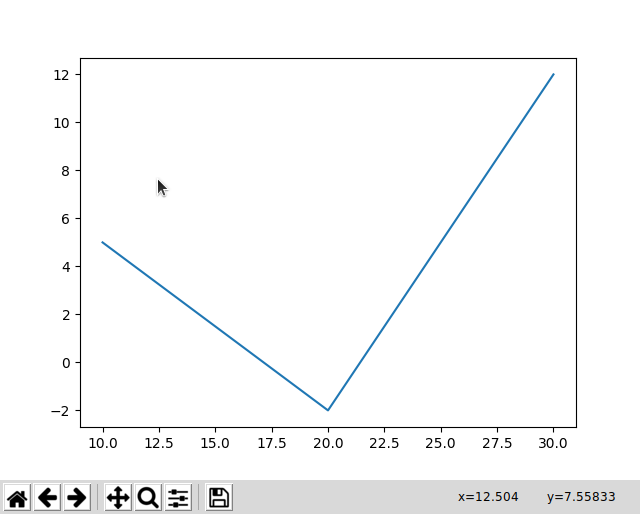### A simple Matplotlib plot

CIAO provides support for the TkAgg and Agg backends. The figure here shows the display from the TkAgg backend (it is missing the window display, which depends on the OS you are using), and includes some basic GUI elements.

These GUI buttons let you scroll and zoom around the display and create a "hardcopy" version of the plot, but do not have the full functionality of the ChIPS GUI.

There are two problems with using show that make it unsatisfactory for an interactive session:

1. You are unable to enter any more commands at the IPython prompt until the window is closed;

2. once closed, the plot can not be reshown or changed (i.e. using plt.show() will not re-display the plot).

### Using the %matplotlib "magic" command

Before calling any plotting command, use the %matplotlib "magic" command:

>>> %matplotlib
Using matplotlib backend: TkAgg


This will work in an IPython session, as well as Sherpa and ChIPS sessions (which are just "wrappers" or "skins" around IPython). CIAO 4.11 comes with the TkAgg Matplotlib interactive backend, and the Agg non-interactive ("hardcopy") backend.

After this call, plots will appear as the calls are made, and can be adjusted. For example (the output from these functions are not shown in the following):

>>> plt.plot([10, 50, 200], [40, 60, 20], 'ko')
>>> plt.xscale('log')
>>> plt.xlabel('The X axis')
>>> plt.ylabel('The exciting Y axis')
>>> plt.savefig('exciting.png')


displays the plot after each call, and the output is shown in the figure below:

### A slightly-exciting Matplotlib plot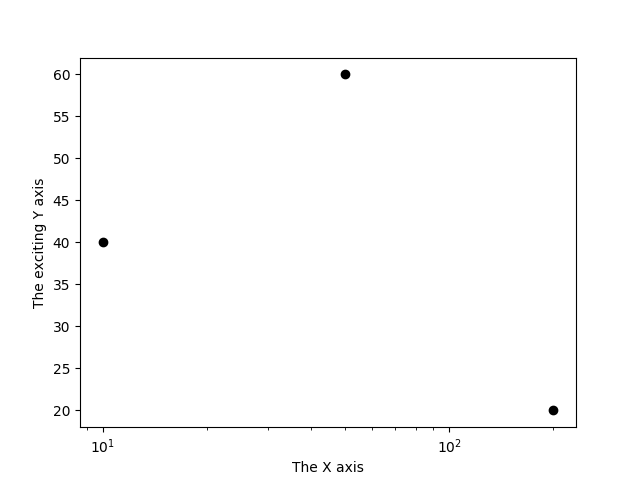### A slightly-exciting Matplotlib plot

As this is the output of the savefig call, the plot does not contain the GUI elements seen in the previous figure.

### Using the %matplotlib command in a Jupyter notebook

When used in a Jupyter notebook, the "inline" or "notebook" option should be used, to make sure the figures are displayed as part of the cell output. That is, you use:

In : %matplotlib inline


The difference between "inline" and "notebook" is that the former displays the PNG output as the cell output, whereas the notebook version displays a single interactive version (similar to that seen when using IPython interactively).

### Using the --matplotlib command-line argument

IPython (but not Sherpa or ChIPS) can be started with the --matplotlib command-line option, which is equivalent to %matplotlib magic command.

unix% ipython --matplotlib
Python 3.5.4 (default, Sep 14 2018, 15:42:52)
Type 'copyright', 'credits' or 'license' for more information
IPython 6.5.0 -- An enhanced Interactive Python. Type '?' for help.
Using matplotlib backend: TkAgg

In :


## Adjusting an existing plot

By default Sherpa will use ChIPS to create plots, but it can be changed to use Matplotlib by changing the setting of the plot_pkg option in the $HOME/.sherpa.rc resource file (this is read when the Sherpa module is first loaded, so can not be changed for a running Sherpa session). The commands used to create the Sherpa plots - such as plot_data and plot_fit_resid - as well as those that change the Sherpa configuration - such as set_xlog - are independent of the plotting backend, but once the plot has been created they are manipulated with commands from the backend. This section provides a quick guide to help users switch from using ChIPS to Matplotlib commands for adjusting existing plots. The savefig function creates a hardcopy version of the plot. For example >>> plt.savefig('plot.png') The set of output formats depends on the backend, with the TkAgg supporting: eps, pdf, pgf, png, ps, raw, rgba, svg, and svgz. The set of options for controlling the ouput are similar to, but not identical, to print_window.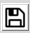The plot window also contains a "Save the figure" button which lets you chose the location and type of the output. The scaling of an axis can be changed with the xscale and yscale functions. These functions also provide limited functionality to change the axis display, such as the number of minor tick marks. The following will change the Y axis to display a logarithmic scale: >>> plt.yscale('log') The limits of an axis can be found or changed with the xlim and ylim functions. Calling with no arguments returns the current limits: >>> ylo, yhi = plt.ylim() Calling with arguments will change the limits, and return the new limits. In the following, the X range is changed to 0 to 25 and the minimum Y value is changed to 0.1 (note that the screen output of these commands is not shown): >>> plt.xlim(0, 25) >>> plt.ylim(ymin=0.1)  The labels of a plot can be changed with the xlabel, ylabel, and title functions. The following changes the X-axis label: >>> plt.xlabel('Energy (keV)')  Note that Matplotlib has more-extensive LaTeX emulation capabilities than ChIPS, and the means of controling the font properties are different. The positioning of a plot can be adjusted with the subplots_adjust function. The following changes the left margin of the plot: >>> plt.subplots_adjust(leftmargin=0.2)  Note that although ChIPS and Maplotlib use a "fractional" coordinate system for the plot margins (the values range between 0 and 1), the values are not going to be identical (as the plot elements are not guaranteed to be the same size). Minor adjustment to the coordinates is therefore likely to be needed when converting from ChIPS to Matplotlib.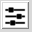The plot window also contains a "Configure subplots" button which lets you select the margin widths using the GUI. The clf function will remove any plots from an existing window. >>> plt.clf() The close function will close one or more plot windows. For example >>> plt.close() ## Changing the appearance of data In ChIPS, properties of displayed data - such as the symbol style of a curve or the line style used by a histogram - can be changed by specifying the name of the item in the appropriate call, such as: >>> set_curve(['symbol.style', 'square', 'symbol.fill', True]) >>> set_histogram('hist2', ['line.style', 'shortdash'])  where the first call changes the current curve, and the second one changes the histogram with the label 'hist2'. The Matplotlib approach is to use objects to represent the plot items, with methods of the objects used to query and change the display properties. These objects are similar to ChIPS concepts - such as axes, curves, and images - but are not identical. The objects are returned when the data is added to the plot, or can be retrieved from the plot to which they were added. In this section we will concentrate on how to change the properties of an existing plot. The first step is to use the gca function to return the current pair of axes: >>> ax = plt.gca()Note Note that this function will create a plot if one does not exist. Many plots are represented by a Matplotlib Line2D object, which can be retrieved with the get_lines method. The following example uses a Sherpa plot_fit plot as a start: >>> set_xlog() >>> set_ylog() >>> plot_fit()  ### A Sherpa plot (before modification)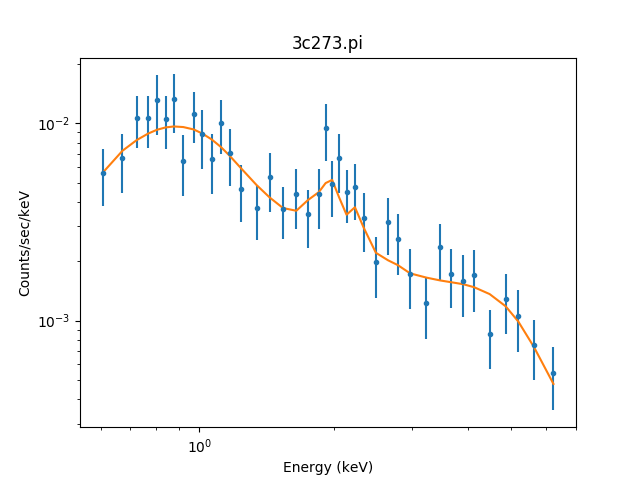### A Sherpa plot (before modification) The output of the plot_fit call, where the Sherpa set_xlog and set_ylog functions where used to set both axes to use a logarithmic scale. In this example the get_lines method returns three lines: >>> ax = plt.gca() >>> print(ax.get_lines()) <a list of 3 Line2D objects> >>> lines = ax.get_lines()  The Line2D class contains many methods to query and change the properties. We first use get_color and get_linestyle to find out some information on these lines: >>> print([l.get_color() for l in lines]) ['#1f77b4', '#1f77b4', '#ff7f0e'] >>> print([l.get_linestyle() for l in lines]) ['-', 'None', '-'] >>> print([l.get_marker() for l in lines]) ['None', '.', 'None'] >>> print([l.get_visible() for l in lines]) [False, True, True]  The output suggests that the first two elements represent the data (the first is for a line connecting the points, which by default is not shown, and the second the symbols at the points), and the last elements represents the fit. Given this, we create variables to represent the "line", "symbol", and "line" parts of the "data" and "fit" plots: >>> ldata, sdata, lfit = lines  The visibility of the line can be changed with set_visible: >>> ldata.set_visible(True) >>> ldata.set_visible(False)  There are a variety of symbol styles, called marker styles in Matplotlib. The following changes from the point ('.') style to a square using set_marker: >>> sdata.set_marker('s')  The fit line is changed to a thick black line, and made slightly transparent, with the following function calls: >>> lfit.set_color('black') >>> lfit.set_linewidth(4) >>> lfit.set_alpha(0.6)  The axes object can also be used to change other parts of the plot, for example the plot title: >>> ax.set_title('A modified plot')  ### A Sherpa plot (after modification)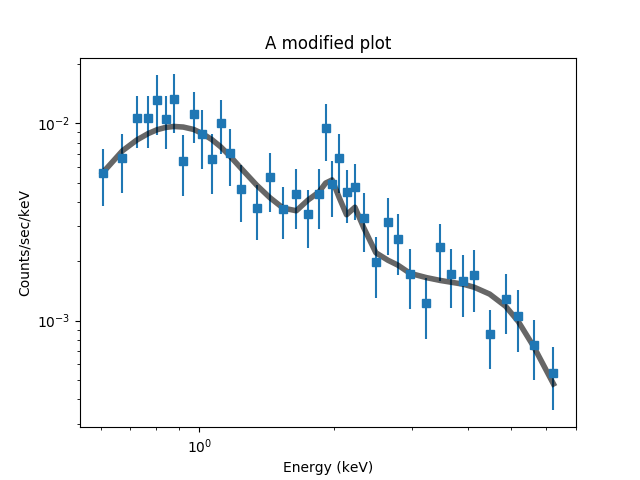### A Sherpa plot (after modification) The Matplotlib calls have modified the plot from the original version. Images can be retrieved with the get_images method. ### Line styles The 'Line Style' section of ahelp chipsopt described the line styles supported by ChIPS, and the Matplotlib styles are described in the Line2D documentation. The following table gives a basic conversion between the two, but the conversion is not exact so some line styles may need changing (in the table below the same Matplotlib option is used to represent several ChIPS options). ChIPS line style Matplotlib option 'noline', 'none' '', ' ', or 'None' 'solid' '-' or 'solid' 'dot' ':' or 'dotted' 'dotlongdash' '-.' or 'dashdot' 'dotshortdash' '-.' or 'dashdot' 'longdash' '--' or 'dashed' 'shortdash' '--' or 'dashed' 'shortdashlongdash' use a "dash-tuple" to create the pattern For complicated scenarios it is possible to define your own spacing and length of dots and dashes in Matplotlib using a "dash tuple", as described in the Matplotlib documentation. The set_drawstyle function can be used to change how points are connected (e.g. straight line or stepped). ### Symbol styles The 'Symbol Style' section of ahelp chipsopt described the symbol styles supported by ChIPS, and there is also the ability to change the angle, size, and fill style of the symbols. In Matplotlib, symbols are referred to as markers, and as with the line styles there is not always a one-to-one correspondance with ChIPS. ChIPS symbol style Matplotlib option 'none' '', ' ', or 'None' 'cross' 'x' or 'X' 'diamond' 'd' or 'D' 'downtriangle' 'v' or '1' 'circle' 'o' 'plus' '+' or 'P' 'square' 's' 'uptriangle' '^' or '2' 'point' '.' 'arrow' Please see the Matplotlib documentation Note that Matplotlib supports more symbols than ChIPS, including the ability to define your own and using text. Matplotlib also allows you to distinguish the fill (face) color from the edge color of a symbol. ## Displaying data The main functions for plotting data in Matplotlib are plot, for one-dimensional data (i.e. x and y values), and imshow for two-dimensional (image) data, but there are a number of other functions, such as scatter and hist. Two major changes to ChIPS are: • there is no equivalent to the "default" color setting, since the same foreground and background colors are used when displaying to the screen or to a hardcopy format; • and Matplotlib will cycle through colors when displaying multiple data sets in a plot, unless the colors are explicitly set. The call to use depends on how the data is to be displayed. The following examples use: >>> import numpy as np >>> x = np.arange(-2, 2, 0.1) >>> ysin = np.sin(x) >>> ycos = np.cos(x)  ### Lines and no points The following plots the sine curve as a solid blue line and the cosine curve as a dotted orange curve. >>> plt.clf() >>> plt.plot(x, ysin, '-') >>> plt.plot(x, ycos, ':')  ### A curve drawn with only lines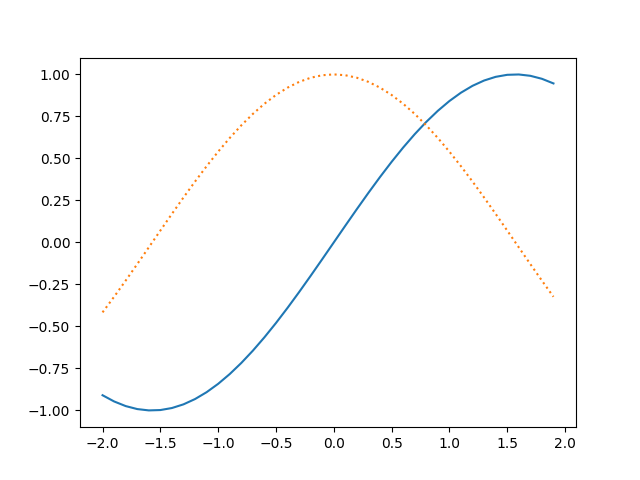### A curve drawn with only lines The set_linestyle function describes the supported list of line styles. ### Points and no lines This time the plot function is used to draw symbols: the sine curve as points and the cosine curve as circles. The output of the plot call is shown below to highlight the fact that it creates Line2D objects. >>> plt.clf() >>> plt.plot(x, ysin, '.') [<matplotlib.lines.Line2D at 0x7faac46df630>] >>> plt.plot(x, ycos, 'o') [<matplotlib.lines.Line2D at 0x7faac598d7b8>]  ### A curve drawn with only symbols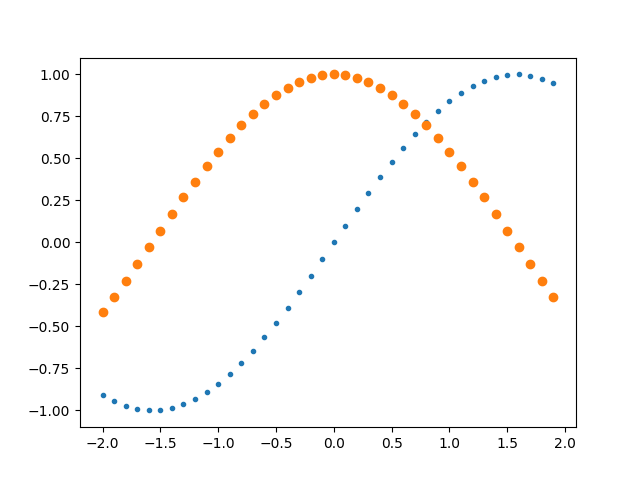### A curve drawn with only symbols Note that the colors for the two lines match those for the previous plot, since the color cycling uses the same sequence after the plot has been cleared with clf. The scatter function could also have been used to create this plot. For example, the following commands create the same plot as previously: >>> plt.clf() >>> plt.scatter(x, ysin, marker='.') >matplotlib.collections.PathCollection at 0x7faac46d5f98> >>> plt.scatter(x, ycos, marker='o') <matplotlib.collections.PathCollection at 0x7faac46c76a0>  Note that the scatter function has different parameter order and names than plot, and it returns a single PathCollection object rather than a list of Line2D objects. In this example the functionality of plot and scatter appear very similar, but they both have their strengths. For example, scatter can vary both the symbol and color for each point (e.g. the radius of a circle and its color can be used to support showing four values per point instead of just two). ### Lines and points The plot function can be used to create both symbols at each point and a line connecting the points. Continuing our example, we have: >>> plt.clf() >>> plt.plot(x, ysin, 'o-') [<matplotlib.lines.Line2D at 0x7faac46a2198>] >>> plt.plot(x, ycos, 'p-.') [<matplotlib.lines.Line2D at 0x7faac4673630>]  ### For when symbols and lines are best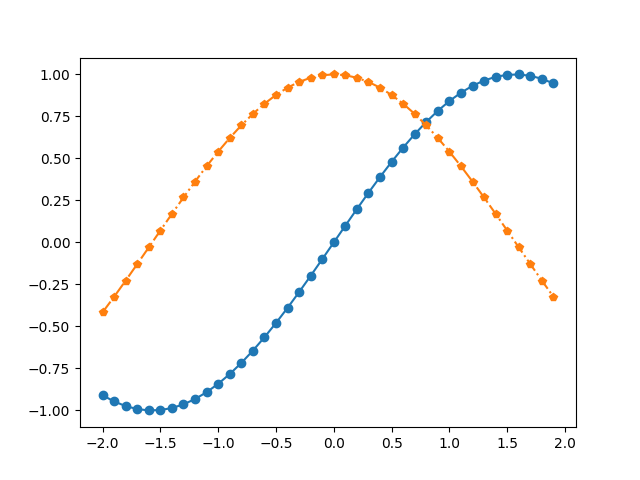### For when symbols and lines are best The line and symbol options have been changed slightly from previous examples: in this case the cosine curve is marked with pentagons and a dot-dashed line (although the symbol size and spacing of the symbols makes it hard to make out the line pattern). Support for drawing histograms is significantly different in Matplotlib to ChIPS, since the basic Matplotlib call creates the histogram and displays it, whereas ChIPS requires a pre-binned dataset. The following examples use the following normally-distributed set of points, with a mean of 1000 and a standard deviation of 150: >>> import numpy as np >>> np.random.seed(238253) >>> v = np.random.normal(loc=1000, scale=150, size=1000)  ### The basic histogram The hist function will bin the data and then display it, while also returning the binned data along with objects for each histogram bin. The default behavior is to chose 10 equally-spaced bins, as shown below: >>> plt.hist(v) (array([ 1., 8., 62., 170., 312., 284., 114., 42., 6., 1.]), array([ 422.09393847, 540.40198572, 658.71003297, 777.01808022, 895.32612747, 1013.63417472, 1131.94222197, 1250.25026922, 1368.55831647, 1486.86636372, 1605.17441097]), <a list of 10 Patch objects>)  ### The default one-dimensional histogram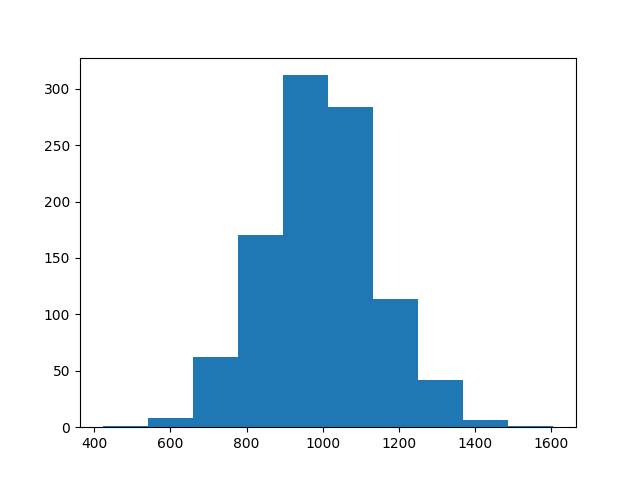### The default one-dimensional histogram The default behavior is to draw filled bins for the histogram. The return value from hist contains the Y values (10 values), edges of the bins (11 values), and then a list of Matplotlib objects, one for each bin (so 10 values). ### Changing the binning The range and bins arguments can be used to change how the input data is binned. In this case we are going to use 20 regularly spaced bins between 400 and 1600, but an array of bin edges can be used for those cases where irregular bins are required. The edgecolor and facecolor arguments are used to change the appearance of the histogram (these are "patch properties"). >>> plt.clf() >>> plt.hist(vrange=(400,1600), bins=20, edgecolor='orange', facecolor='none')) (array([ 1., 0., 1., 4., 16., 36., 64., 89., 131., 163., 182., 129., 77., 51., 35., 12., 8., 0., 0., 0.]), array([ 400., 460., 520., 580., 640., 700., 760., 820., 880., 940., 1000., 1060., 1120., 1180., 1240., 1300., 1360., 1420., 1480., 1540., 1600.]), <a list of 20 Patch objects>)  ### Increasing the bin count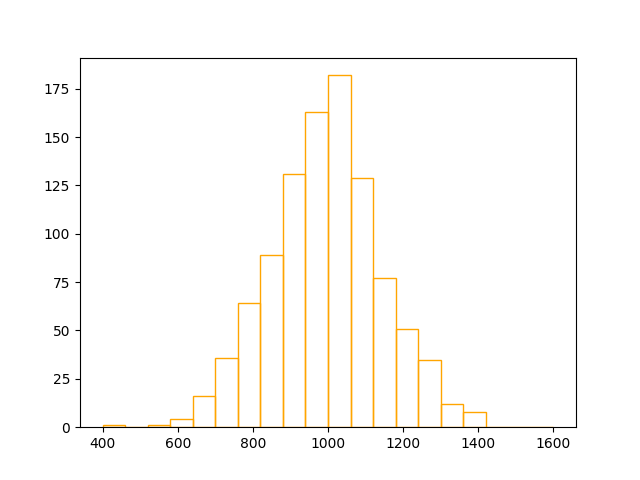### Increasing the bin count ### Plotting up pre-binned data If the data has already been pre-binned, that is you have the edge values and the values per bin, as returned by NumPy's histogram routine here but probably read in from a file or computed by some other routine in a real-world situation: >>> y, edges = np.histogram(v, bins=20, range=(400, 1600))  then the fill_between function can be used to flood the area below the points, effectively creating a histogram. Note that the bin values array needs to be increased by adding a 0 on the start, which is done with the NumPy concatenate function. >>> y0 = np.concatenate((, y)) >>> plt.fill_between(edges0, y, step='pre', edgecolor='orange', alpha=0.8)  This suggestion is based on a StackOverflow answer. ### Plotting pre-binned data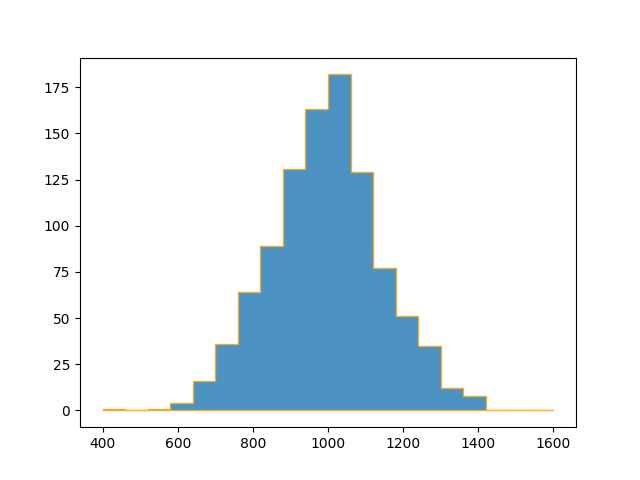### Plotting pre-binned data The resulting plot is similar to previous versions, but note that the default choice for the Y-axis range extends below zero, and the edges of each bin do not extend down to zero, unlike the previous example. The contour and contourf functions plot contours and filled contours respectively (ChIPS does not support filled contours). The following example uses an image from the CIAO smoke test suite (the background estimated by wavdetect), which will be read in using Crates, with the pixel values copied into a NumPy array called imgvals: >>> import os >>> import pycrates >>> infile = os.environ['ASCDS_INSTALL'] + '/test/smoke/data/tools-wav1_bkg.fits' >>> cr = pycrates.read_file(infile) >>> imgvals = cr.get_image().values >>> print(imgvals.shape) (334, 334)  The Matplotlib image routines default to placing the image origin in the top-left corner of the plot, whereas ChIPS uses the bottom-left (matching the DS9 display). The origin argument can be set to lower to change this: >>> plt.clf() >>> plt.contour(imgvals, origin='lower') <matplotlib.contour.QuadContourSet at 0x7fe6711a27b8>  ### The default contour plot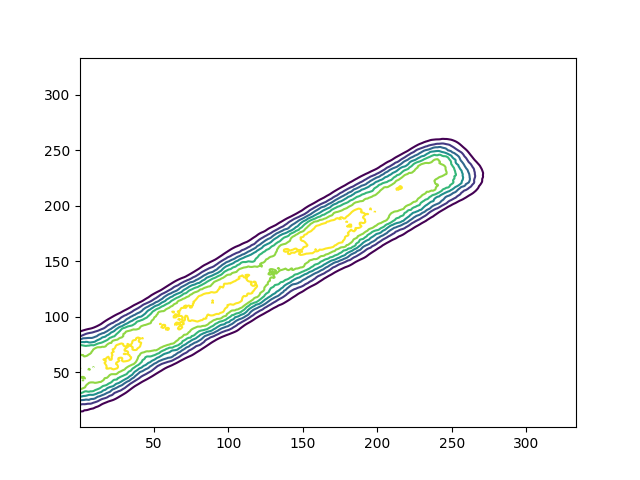### The default contour plot Unlike ChIPS, Matplotlib defaults to creating contours with different colors for the levels. Labels can be added using the clabel function, which requires saving the return value from contour: >>> plt.clf() >>> contours = plt.contour(imgvals, levels=[0.2, 0.6, 1.0], origin='lower') >>> plt.clabel(contours) <a list of 4 text.Text objects>  ### Specifying and labelling levels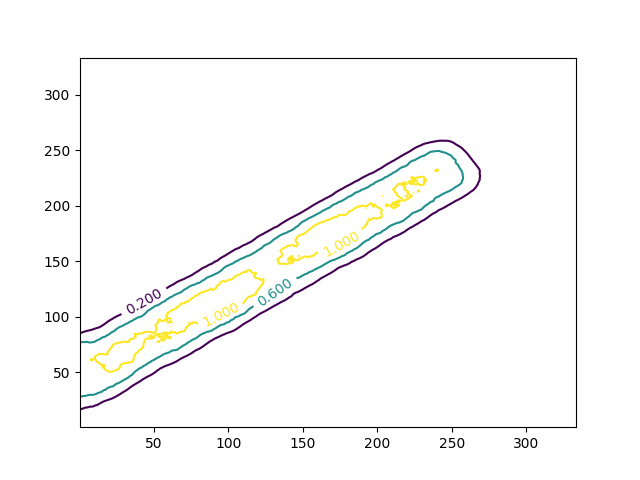### Specifying and labelling levels Note that ChIPS does not support labelling contours. Contours can be overlain on existing plots (here we overlay a coarse grid of contours on a finer grid of filled contours and ensure the axes use the same number of pixels for the same data range): >>> plt.clf() >>> plt.contourf(imgvals, origin='lower') >>> plt.contour(imgvals, levels=[0.2, 0.6, 1.0], origin='lower', colors='white') >>> plt.axis('equal')  ### Filled contours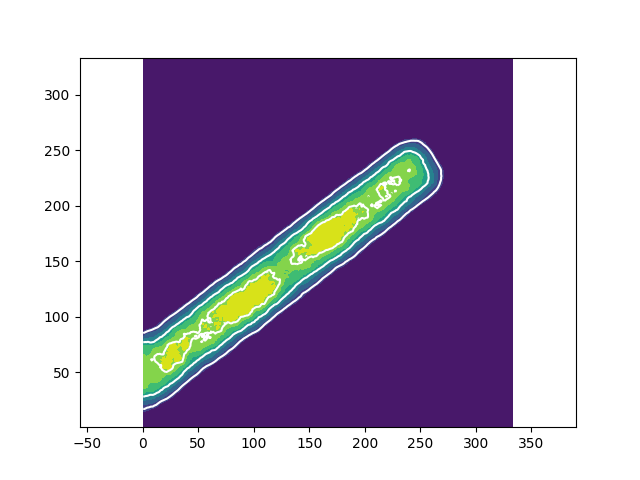### Filled contours Note that ChIPS does not directly support filled contours, although it can be emulated with multiple calls to add_region. The axis function provides a number of functions; here we use it to set the aspect ratio of the plot (i.e. the number of pixels used to represent a change of 50 units is the same for both axes). Since the default window size has more space horizontally than vertically but the data that is displayed is square, the axes are expanded along the X axis compared to the previous version of the plot. This could also have been achieved using the "object-oriented" interface (which can be mixed-and-matched with the "pyplot" functions) by saying: >>> ax = plt.gca() >>> ax.set_aspect('equal', 'datalim')  Here the gca call is used to get the plot axes, so that they can be changed to use the same scale for both axes with the set_aspect call. The imshow and colorbar routines display images and color bars in Matplotlib. As with contours, the origin parameter needs to be set to match the orientation used by ChIPS. The following examples use the same imgvals data as above. >>> plt.clf() >>> plt.imshow(imgvals, origin='lower') <matplotlib.image.AxesImage at 0x7fe6705c3e48> >>> plt.colorbar() <matplotlib.colorbar.Colorbar at 0x7fe6705fbc50>  ### The default image and colorbar display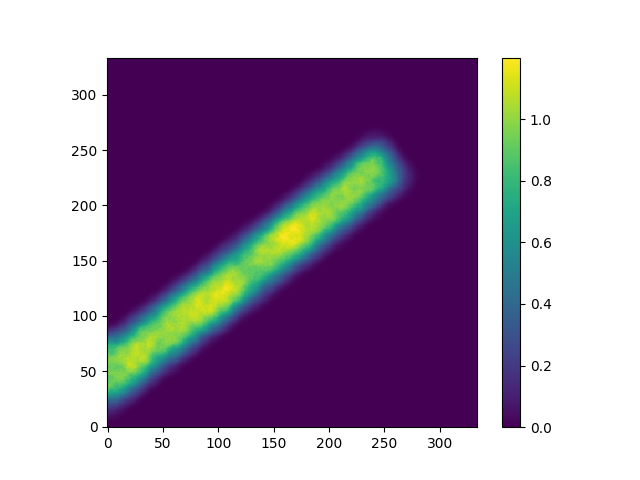### The default image and colorbar display The default colormap depends on the version of Matplotlib. For 2.2.3, provided with CIAO 4.11, this is viridis. There are a wide variety of options provided by Matplotlib for displaying images. For example, the scaling used to map the pixel values to a color can be changed from a linear scale to a variety of options - so called "Colormap Normalization" - such as a logarithmic (base 10) scale, as shown below. The scaling needs to know the minimum and maximum values to use, which for this image is roughly 0 to 1.2: >>> print(imgvals.min()) 0.0 >>> print(imgvals.max()) 1.19966  Since the minimum value has to be positive for a logarithm, we chose 0.1: >>> from matplotlib import colors >>> lnorm = colors.LogNorm(vmin=0.1, vmax=1.2)  The normalization object can now be given as the norm argument of the imshow call to apply the scaling: >>> plt.clf() >>> plt.imshow(imgvals, origin='lower', norm=lnorm) >>> plt.colorbar()  ### Using a log scale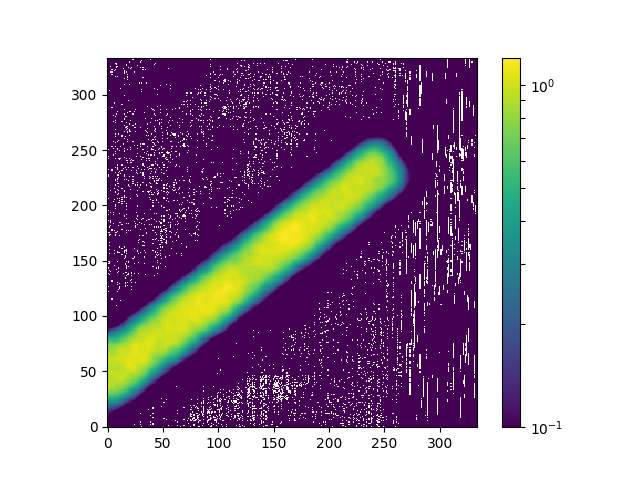### Using a log scale As the image does not have a large dynamic range the use of a logarithmic scale does not add much to the linear display used previously. The white pixels in the background are those which are invalid after applying the scaling (i.e. those pixels in the input which have a zero value). The colorbar is drawn using a logarithmic scale. Note that ChIPS does not provide this level of flexibility, since the image scaling has to be applied to the pixel values sent to the add_image call, and the color bar only ever displays a linear scale. The text function is used to add labels to plots. The Matplotlib introduction to text documentation should be reviewed to see what capabilities Matplotlib has. For example, the following will add the unimaginative label "A label" to the plot starting at 100, 250, colored orange and with a size of 14. >>> plt.text(100, 250, 'A label', color='orange', fontsize=14)  As with ChIPS, Matplotlib has support for LaTeX commands, changing the font style, location of the text with respect to the given coordinate, and a number of other options. The figure function is used to create a new window in which to display plots. Note that, unlike add_window, plt.figure returns an object which can be used to change the properties of the display. This object can also be retrieved with the gcf function. A comparison of ChIPS and Matplotlib with their default arguments: >>> pychips.add_window() >>> plt.figure() <Figure size 640x480 with 0 Axes>  and with explicit window sizes (the units for the figsize argument is inches): >>> pychips.add_window(11, 8, 'inches') >>> plt.figure(figsize=(11, 8)) <Figure size 1100x800 with 0 Axes>  Both ChIPS and Matplotlib support complicated plot arrangements (as discussed in the following section), including support for plots with multiple axes. Although the systems are not identical (in that ChIPS allows adding individual axes whereas Matplotlib works with pairs of axes), a common case is adding a second Y axis to a plot, which can be handled with twinx function. As an example, consider plotting against time the ra and dec columns of the aspect solution file$ASCDS_INSTALL/test/smoke/data/pcadf141725632N002_asol1.fits (it is one of the files provided as part of the CIAO smoke test suite):

>>> import os
>>> ciaodir = os.getenv('ASCDS_INSTALL')
>>> indir = os.path.join(ciaodir, 'test', 'smoke', 'data')
>>> infile = os.path.join(indir, 'pcadf141725632N002_asol1.fits')
>>> cr = pycrates.read_file(infile)
>>> ra = cr.get_column('RA').values
>>> dec = cr.get_column('Dec').values
>>> time = cr.get_column('time').values


With this, the two curves can be plotted on the same plot by adding a second Y axis for the declination data using ChIPS,

>>> pychips.erase()
>>> pychips.add_curve(time, ra, ['symbol.style', 'none'])
>>> pychips.set_plot_xlabel('Time')
>>> pychips.set_plot_ylabel('RA')
>>> pychips.add_axis(pychips.Y_AXIS, 1, 0, 1)
>>> pychips.add_curve(time, dec, ['*.color', 'orange', 'symbol.style', 'none'])
>>> pychips.set_plot_ylabel('Dec')
>>> pychips.set_plot(['rightmargin', 0.15])


which creates the following figure:

### Adding a second Y axis in ChIPS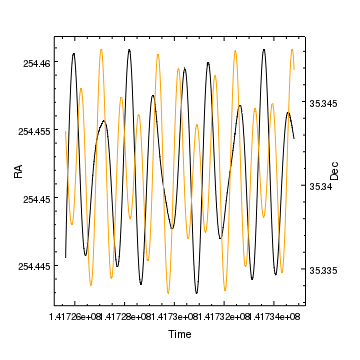### Adding a second Y axis in ChIPS

Since this example is primarily about adding an extra axis, no attempt has been made to change the axis labels and tick marks.

The equivalent Matplotlib version is:

>>> plt.clf()
>>> plt.plot(time, ra)
>>> plt.xlabel('Time')
>>> plt.ylabel('RA')
>>> ax1 = plt.gca()
>>> ax2 = plt.twinx()
>>> plt.plot(time, dec, color='orange')
>>> plt.ylabel('Dec')
>>> plt.subplots_adjust(right=0.85)


which creates the Matplotlib version:

### Re-creating the add_axis plot in Matplotlib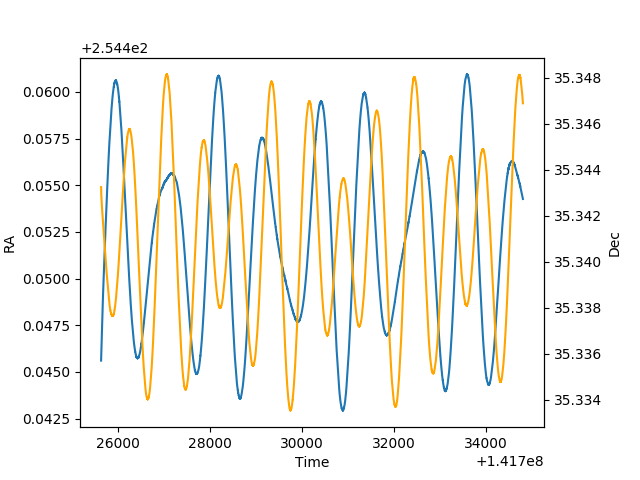### Re-creating the add_axis plot in Matplotlib

The color for the RA data values is blue (the default color used by Matplotlib) rather than black (the default ChIPS color for hardcopy output).

The Data Science Handbook provides a good introduction to Matplotlib's support for multiple plots in a figure. Below we show how several common ChIPS-style plot arrangements can be created in Matplotlib.

### The split command

The closest to split is the subplot function, except that split will create plots (with no axes) whereas as subplot will only create a plot (pair of axes) for the requested plot number (the third argument to the call). So, after

>>> pychips.clear()
>>> pychips.split(3, 2)
>>> pychips.add_axis(pychips.XY_AXIS, 0, 0, 1)
>>> pychips.current_plot('plot4')
>>> pychips.add_axis(pychips.X_AXIS, 0, 10, 20)
>>> pychips.add_axis(pychips.Y_AXIS, 0, 1000, 2000)


and

>>> plt.clf()
>>> plt.subplot(3, 2, 1)
<matplotlib.axes._subplots.AxesSubplot at 0x7f423cd3e3c8>
>>> plt.subplot(3, 2, 4)
<matplotlib.axes._subplots.AxesSubplot at 0x7f4238574d30>
>>> plt.xlim(10, 20)
(10, 20)
>>> plt.ylim(1000, 2000)
(1000, 2000)


the windows look somewhat different.

### Comparing split to subplot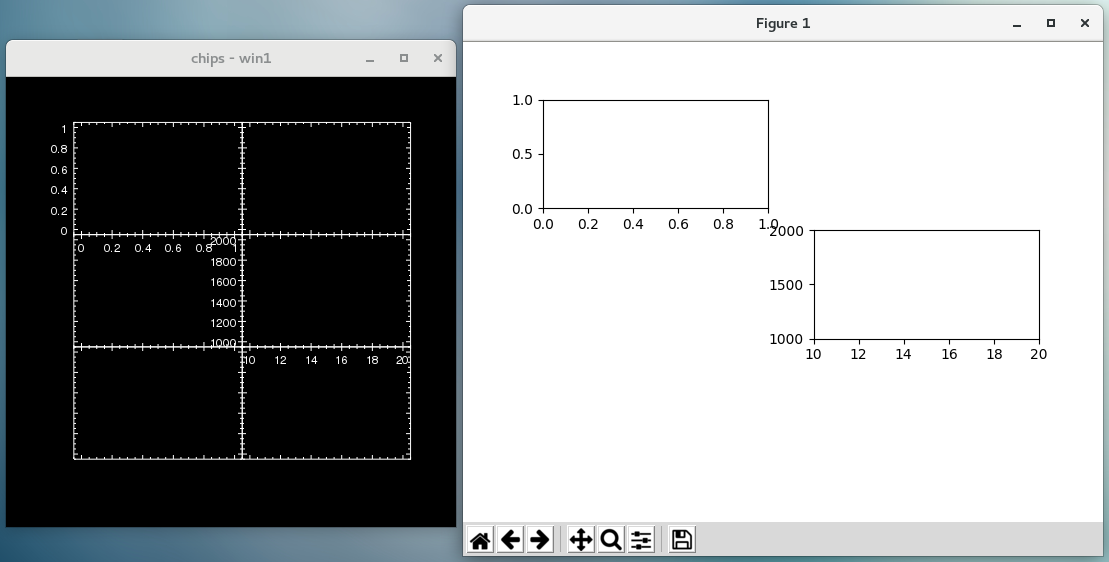### Comparing split to subplot

ChIPS has created plot areas for all 6 grid elements, whereas Matplotlib has just created a plot (and axes) for the first and fourth elements of the grid (both count from left to right, top to bottom).

The spacing between the plots in ChIPS can be adjusted either in the grid call (as optional arguments), or with calls like adjust_grid_gaps (or its variants). The Matplotlib equivalent is subplots_adjust, although the arguments have a different meaning (ChIPS generally works with the spacing between the plots, referred to as the gap), whereas Matplotlib refers to this as the space between the plots (wspace and hspace) which has a different definition to gap. As the sizes of the plot elements and default margins are different in the two systems, some trial and error will be required when converting between the two.

### The strip_chart command

The strip_chart ChIPS command creates a number of vertically-aligned plots, all with a common X axis (or horizontally-aligned with a common Y axis). The Matplotlib subplot family of commands can emulate this with the sharex or sharey argument.

>>> pychips.strip_chart(3)
>>> fig, axes = plt.subplots(3, 1, sharex='col')


### Comparing strip_chart to subplots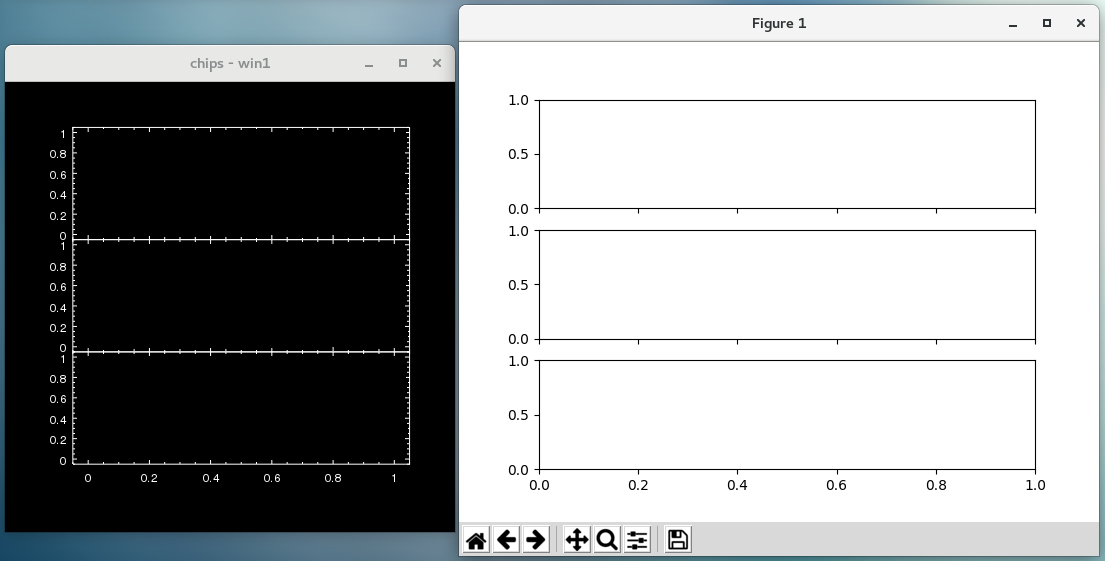### Comparing strip_chart to subplots

As with other commands in this section, the default spacing and arrangement of the plots do not match between ChIPS and Matplotlib.

Another difference is the "current" plot after these calls: ChIPS picks the top plot (which is specialized behavior for strip_chart, as it differs from split), and Matplotlib uses the bottom plot, as shown below:

>>> pychips.add_curve([10, 20, 30], [4000, 3000, 6000])
>>> plt.plot([10, 20, 30], [4000, 3000, 6000], '-x')


### What is the current plot?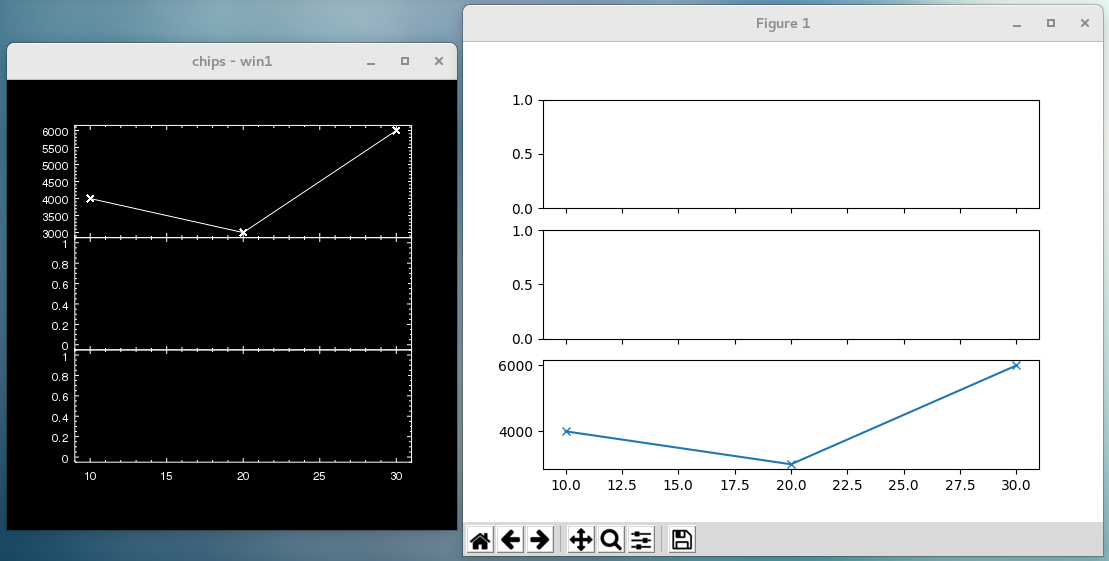### What is the current plot?

The Matplotlib data was plotted using the format of -x to match the default ChIPS behavior of connecting points with a solid line and drawing a cross at each point.

### Complicated grids

If you have created an arrangement of plots which takes advantage of grid_objects, adjust_grid_xrelsize, adjust_grid_yrelsize, adjust_grid_gaps, or one of their variants, then you probably want to use plt.GridSpec to create the grid layout. An example is shown below, but please also see the Please see the Data Science Handbook which provides another example.

>>> pychips.erase()
>>> pychips.split(2, 3, 0.05, 0.05)
>>> pychips.adjust_grid_xrelsizes([1, 2, 1])
>>> pychips.adjust_grid_yrelsize(1, 2)
>>> plt.clf()
>>> grid = plt.GridSpec(3, 4, wspace=0.4, hspace=0.4)
>>> plt.subplot(grid[0:2, 0])
>>> plt.subplot(grid[0:2, 1:3], facecolor='orange')
>>> plt.subplot(grid[0:2, 3], facecolor='teal')
>>> plt.subplot(grid[2, 0], facecolor='firebrick')
>>> plt.subplot(grid[2, 1:3])
>>> plt.subplot(grid[2, 3], facecolor='powderblue')


### A complex arrangement of plots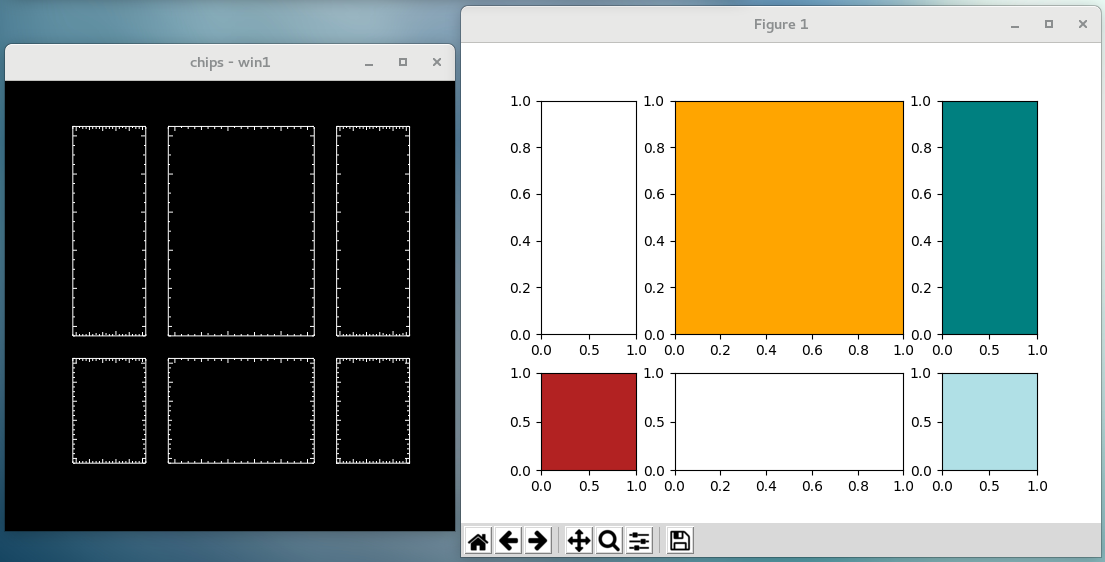### A complex arrangement of plots

Since Matplotlib and ChIPS use a different system for placing and sizing plots and their margins, the two plots are similar but not the same. The colors were added to the Matplotlib plots to help identify the indexing scheme (y and then x) for the grid array with the location on screen.

The make_figure command is a utility routine provided by ChIPS that will read in data, try to recognize the form, and then display it automatically. There is no direct replacement for this in Matplotlib, so you will have to read in the data using Crates and then plot the data using one of the functions described above.

### Plotting curves

As an example, consider plotting the ra and dec columns of the same aspect-solution file as used in the add_axis example above:

>>> import os
>>> ciaodir = os.getenv('ASCDS_INSTALL')
>>> indir = os.path.join(ciaodir, 'test', 'smoke', 'data')
>>> infile = os.path.join(indir, 'pcadf141725632N002_asol1.fits')


With this set up, we can create a figure with ChIPS by saying:

>>> pychips.make_figure(infile + '[cols ra,dec'])


which creates the following figure:

### Plotting a curve with make_figure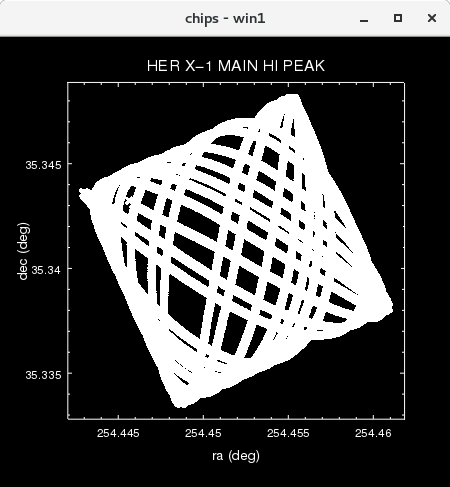[Version: PNG]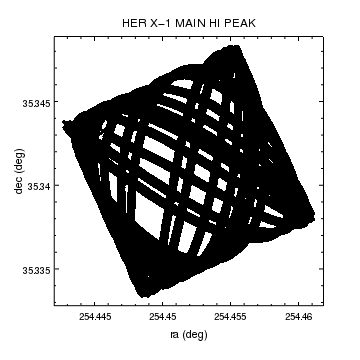### Plotting a curve with make_figure

The make_figure command has read in the RA and Dec values from the file and plotted them against each other. What can not be seen here is that the points have been connected by lines and drawn with a symbol. The Axis units, and plot title, have been read in from the relevant metadata in the file.

The hardcopy version of this plot is available via the PNG link.

An equivalent plot with Matplotlib could be manually recreated with the following commands:

>>> cr = pycrates.read_file(infile + '[cols ra,dec]')
>>> ra = cr.get_column('ra')
>>> dec = cr.get_column('dec')
>>> fig = plt.figure()
>>> plt.plot(ra.values, dec.values, '-x', color='k')
>>> ax = plt.gca()
>>> ax.set_aspect('equal', 'datalim')
>>> plt.xlabel('ra (' + ra.unit + ')')
>>> plt.ylabel('dec (' + dec.unit + ')')
>>> plt.title(cr.get_key_value('OBJECT'))


which creates:

### Re-creating the make_figure plot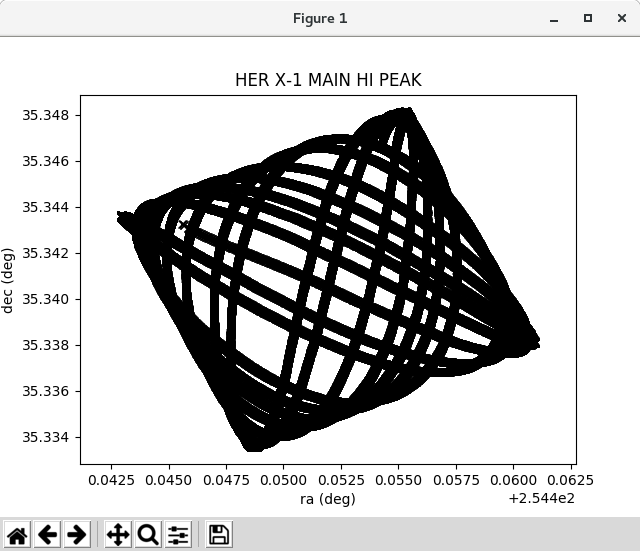[Version: PNG]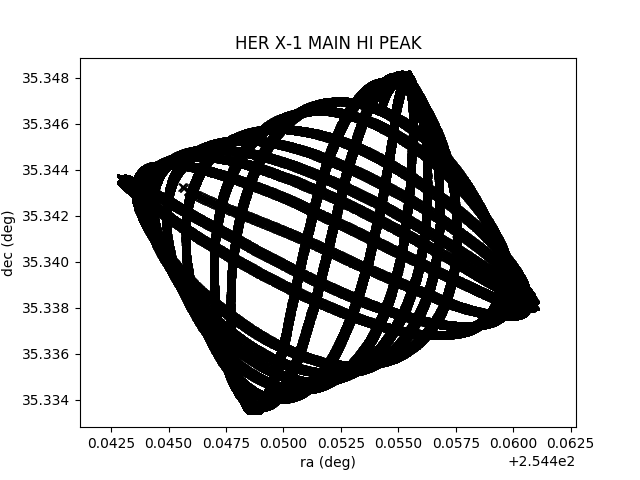### Re-creating the make_figure plot

The Matplotlib calls were made to make the figures similar, and to highlight some of the commands discussed elsewhere on this page. Two things to note with the plt.plot call: the data was plotted in the same way that ChIPS defaults to, in that both symbols at the points and lines connecting them; and the color was explicitly set to black rather than using the default color. The ChIPS plot, when printed out with print_window, switches to using black lines on a white background, whereas Matplotlib uses the same color scheme for the on-screen and hardcopy outputs.

The aspect ratio of the plot is set since at this declination ($$\sim 35^\circ$$) the difference between the two axes is not large.

The hardcopy version of this plot is available via the PNG link.

### Displaying image data with a WCS

There is also support for displaying image data with a World Coordinate System (WCS), but it requires installing Astropy or APLpy into CIAO. The following example uses Astropy, which can be installed with the following:

unix% pip3 install 'astropy<3.1'


The constraint above is to ensure that NumPy is not upgraded. Please see the Python installation guide for more information.

With Astropy installed, we can use Matplotlib to create a figure similar to the following ChIPS commands, which uses the same image as above:

>>> infile = os.environ['ASCDS_INSTALL'] + '/test/smoke/data/tools-wav1_bkg.fits'
>>> pychips.make_figure(infile, 'image')
>>> pychips.set_xaxis(['tickformat', 'dec'])
>>> pychips.set_yaxis(['tickformat', 'dec'])


### Plotting image data with make_figure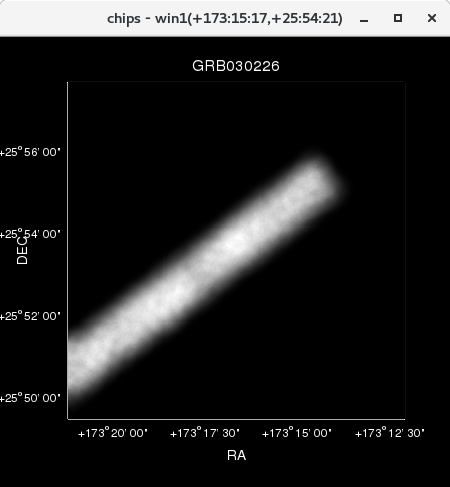[Version: PNG]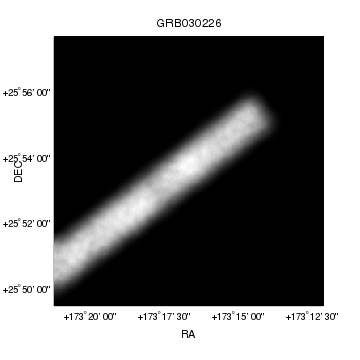### Plotting image data with make_figure

The make_figure command has read in the image pixel values and displayed them, along with the data needed to display the tangent-plane World Coordinate System (the mapping between pixel coordinates and position on the sky). Both axes are labelled as degrees, arcminutes, and arcseconds (rather than having the X axis use hours, minutes, and seconds) to better match the Matplotlib version.

The hardcopy version of this plot is available via the PNG link.

The Matplotlib version requires creating an Astropy WCS object which is used to create a plot with the correct axes. We start by loading in the pixel values and WCS information using crates. The CIAO data model splits the WCS transformation into a logical-to-physical conversion (sky) and a physical-to-equatorial conversion (eqpos), whereas Astropy just wants these to be combined, hence the following steps (there are a number of format conversions used below that are not explicitly called out in the text):

>>> from astropy.wcs import WCS
>>> cr = pycrates.read_file(infile)
>>> imgvals = cr.get_image().values
>>> print(cr.get_axisnames())
['sky', 'EQPOS']
>>> sky = cr.get_transform('sky')
>>> eqpos = cr.get_transform('eqpos')
>>> crpix_sky = eqpos.get_parameter_values'CRPIX')
>>> crpix_log = sky.invert(crpix_sky[np.newaxis, :])
>>> cdelt_sky = eqpos.get_parameter_value('CDELT')
>>> cdelt_log = cdelt_sky * sky.get_parameter_value('SCALE')
>>> crval = eqpos.get_parameter_value('CRVAL')
>>> ctype = [str(v) for v in eqpos.get_parameter_value('CTYPE')]
>>> wcs = WCS(naxis=2)
>>> wcs.wcs.crpix = crpix_log
>>> wcs.wcs.cdelt = cdelt_log
>>> wcs.wcs.crval = crval
>>> wcs.wcs.ctype = ctype


With the WCS object, a plot can be created using this projection, and the image data added to this plot:

>>> fig = plt.figure()
>>> ax = plt.subplot(projection=wcs)
>>> plt.imshow(imgvals, origin='lower')


which creates the following plot:

### Re-creating the make_figure image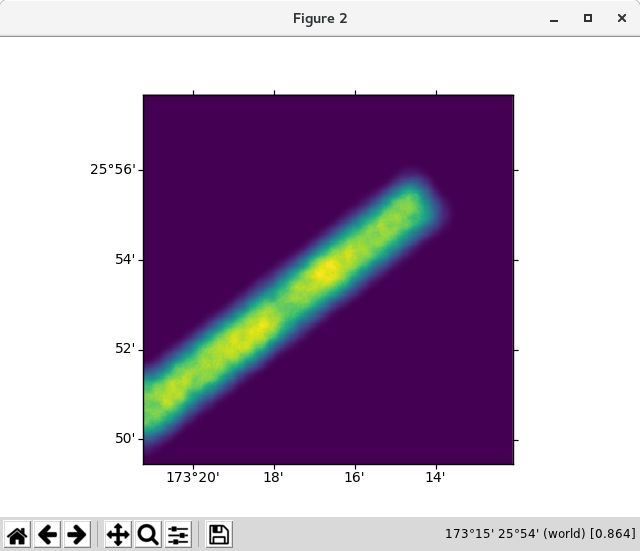[Version: PNG]### Re-creating the make_figure image

The default display for the axes is to use degrees and minutes, which is why the ChIPS version was manipulated to change the tickformat setting, to show that the coordinate values match up.

The hardcopy version of this plot is available via the PNG link.

Note that there are a number of other ways that the Astropy WCS object could have been created, but these are beyond the scope of this document.

## Missing functionality

While Matplotlib has many capabilities that ChIPS does not have, there are some that it does not, including:

• the GUI support in the two systems is very different, with Matplotlib generally lacking the detailed capabilities to edit an existing plot;

• no support for undo and redo;

• no support for "state" files (as used by the save_state and load_state functions;

• support for "bound axes", where changes to the properties of one are automatically propagated to the other, is significantly different in the two systems (e.g. the sharex and sharey arguments of the various subplot functions in Matplotlib);

• the means of identifying what item of the plot to change in Matplotlib does not match the ChIPS concept of currency;

• ChIPS can support multiple clients talking to the same server, which lets users create a plot with prism, edit it with the ChIPS GUI, and also change it from an IPython session.